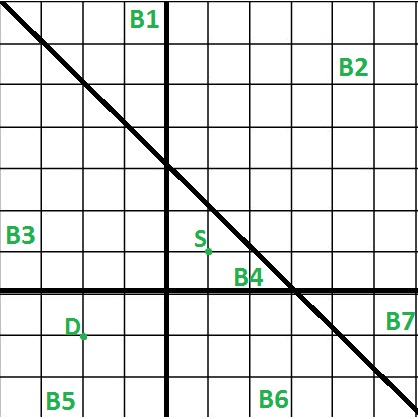Related Articles
Minimum block jumps to reach destination
• Difficulty Level : Medium
• Last Updated : 02 Mar, 2020

Given N lines and one starting point and destination point in 2-dimensional space. These N lines divide the space into some blocks. We need to print the minimum number of jumps to reach destination point from starting point. We can jump from one block to other block only if they share a side.
Examples:

```Input : Lines = [x = 0, y = 0, x + y – 2 = 0]
Start point = [1, 1],
Dest point  = [-2, -1]
Output : 2
We need to jump 2 times (B4 -> B3 then B3 -> B5
or B4 -> B6 then B6 -> B5) to reach destination
point from starting point shown in below diagram.
Each block i is given an id Bi in the diagram.```

## Recommended: Please try your approach on {IDE} first, before moving on to the solution.

We can solve this problem using a property of lines and points which is stated as, If we put two points in line equation then they will have same sign i.e. positive-positive or negative-negative of evaluated value if both points lies on the same side of line, in case of different sign i.e. positive-negative they will lie on different side of line.
Now we can use above property to solve this problem, For each line, we will check if start and destination point lie on the same side or not. If they lie to the different side of a line then that line must be jumped to come closer. As in above diagram start point and the destination point are on the same side of x + y – 2 = 0 line, so that line need not to be jumped, rest two lines need to be jumped because both points lies on opposite side.
Finally, we will check the sign of evaluation of points with respect to each line and we will increase our jump count whenever we found opposite signs. Total time complexity of this problem will be linear.

## C++

 `// C++ program to find minimum jumps to reach``// a given destination from a given source``#include ``using` `namespace` `std;`` ` `// To represent point in 2D space``struct` `point``{``    ``int` `x, y;``    ``point(``int` `x, ``int` `y) : x(x), y(y)``    ``{}``};`` ` `// To represent line of (ax + by + c)format``struct` `line``{``    ``int` `a, b, c;``    ``line(``int` `a, ``int` `b, ``int` `c) : a(a), b(b), c(c)``    ``{}``    ``line()``    ``{}``};`` ` `// Returns 1 if evaluation is greater > 0,``// else returns -1``int` `evalPointOnLine(point p, line curLine)``{``    ``int` `eval = curLine.a* p.x +``               ``curLine.b * p.y +``               ``curLine.c;``    ``if` `(eval > 0)``        ``return` `1;``    ``return` `-1;``}`` ` `//  Returns minimum jumps to reach``//  dest point from start point``int` `minJumpToReachDestination(point start,``              ``point dest, line lines[], ``int` `N)``{``    ``int` `jumps = 0;``    ``for` `(``int` `i = 0; i < N; i++)``    ``{``        ``// get sign of evaluation from point``        ``// co-ordinate and line equation``        ``int` `signStart = evalPointOnLine(start, lines[i]);``        ``int` `signDest = evalPointOnLine(dest, lines[i]);`` ` `        ``// if both evaluation are of opposite sign,``        ``// increase jump by 1``        ``if` `(signStart * signDest < 0)``            ``jumps++;``    ``}`` ` `    ``return` `jumps;``}`` ` `// Driver code to test above methods``int` `main()``{``    ``point start(1, 1);``    ``point dest(-2, -1);`` ` `    ``line lines;``    ``lines = line(1, 0, 0);``    ``lines = line(0, 1, 0);``    ``lines = line(1, 1, -2);`` ` `    ``cout << minJumpToReachDestination(start, dest, lines, 3);`` ` `    ``return` `0;``}`

## Java

 `// Java program to find minimum jumps to reach``// a given destination from a given source``class` `GFG``{`` ` `// To represent point in 2D space``static` `class` `point``{``    ``int` `x, y;`` ` `    ``public` `point(``int` `x, ``int` `y) ``    ``{``        ``super``();``        ``this``.x = x;``        ``this``.y = y;``    ``}``     ` `};`` ` `// To represent line of (ax + by + c)format``static` `class` `line``{``    ``public` `line(``int` `a, ``int` `b, ``int` `c)``    ``{``        ``this``.a = a;``        ``this``.b = b;``        ``this``.c = c;``    ``}`` ` `    ``int` `a, b, c;``     ` `    ``line()``    ``{}``};`` ` `// Returns 1 if evaluation is greater > 0,``// else returns -1``static` `int` `evalPointOnLine(point p, line curLine)``{``    ``int` `eval = curLine.a* p.x +``            ``curLine.b * p.y +``            ``curLine.c;``    ``if` `(eval > ``0``)``        ``return` `1``;``    ``return` `-``1``;``}`` ` `// Returns minimum jumps to reach``// dest point from start point``static` `int` `minJumpToReachDestination(point start,``            ``point dest, line lines[], ``int` `N)``{``    ``int` `jumps = ``0``;``    ``for` `(``int` `i = ``0``; i < N; i++)``    ``{``        ``// get sign of evaluation from point``        ``// co-ordinate and line equation``        ``int` `signStart = evalPointOnLine(start, lines[i]);``        ``int` `signDest = evalPointOnLine(dest, lines[i]);`` ` `        ``// if both evaluation are of opposite sign,``        ``// increase jump by 1``        ``if` `(signStart * signDest < ``0``)``            ``jumps++;``    ``}`` ` `    ``return` `jumps;``}`` ` `// Driver code ``public` `static` `void` `main(String[] args)``{``    ``point start = ``new` `point(``1``, ``1``);``    ``point dest = ``new` `point(-``2``, -``1``);`` ` `    ``line []lines = ``new` `line[``3``];``    ``lines[``0``] = ``new` `line(``1``, ``0``, ``0``);``    ``lines[``1``] = ``new` `line(``0``, ``1``, ``0``);``    ``lines[``2``] = ``new` `line(``1``, ``1``, -``2``);`` ` `    ``System.out.print(minJumpToReachDestination(start, dest, lines, ``3``));``}``}`` ` `// This code is contributed by Rajput-Ji`

## C#

 `// C# program to find minimum jumps to reach``// a given destination from a given source``using` `System;`` ` `class` `GFG``{`` ` `// To represent point in 2D space``class` `point``{``    ``public` `int` `x, y;`` ` `    ``public` `point(``int` `x, ``int` `y) ``    ``{``        ``this``.x = x;``        ``this``.y = y;``    ``}``     ` `};`` ` `// To represent line of (ax + by + c)format``class` `line``{``    ``public` `int` `a, b, c; ``    ``line()``    ``{}``    ``public` `line(``int` `a, ``int` `b, ``int` `c)``    ``{``        ``this``.a = a;``        ``this``.b = b;``        ``this``.c = c;``    ``}``};`` ` `// Returns 1 if evaluation is greater > 0,``// else returns -1``static` `int` `evalPointOnLine(point p, line curLine)``{``    ``int` `eval = curLine.a* p.x +``            ``curLine.b * p.y +``            ``curLine.c;``    ``if` `(eval > 0)``        ``return` `1;``    ``return` `-1;``}`` ` `// Returns minimum jumps to reach``// dest point from start point``static` `int` `minJumpToReachDestination(point start,``            ``point dest, line []lines, ``int` `N)``{``    ``int` `jumps = 0;``    ``for` `(``int` `i = 0; i < N; i++)``    ``{``        ``// get sign of evaluation from point``        ``// co-ordinate and line equation``        ``int` `signStart = evalPointOnLine(start, lines[i]);``        ``int` `signDest = evalPointOnLine(dest, lines[i]);`` ` `        ``// if both evaluation are of opposite sign,``        ``// increase jump by 1``        ``if` `(signStart * signDest < 0)``            ``jumps++;``    ``}`` ` `    ``return` `jumps;``}`` ` `// Driver code ``public` `static` `void` `Main(String[] args)``{``    ``point start = ``new` `point(1, 1);``    ``point dest = ``new` `point(-2, -1);`` ` `    ``line []lines = ``new` `line;``    ``lines = ``new` `line(1, 0, 0);``    ``lines = ``new` `line(0, 1, 0);``    ``lines = ``new` `line(1, 1, -2);`` ` `    ``Console.Write(minJumpToReachDestination(start, dest, lines, 3));``}``}`` ` `// This code is contributed by Rajput-Ji`

Output:

```2
```

This article is contributed by Utkarsh Trivedi. If you like GeeksforGeeks and would like to contribute, you can also write an article using contribute.geeksforgeeks.org or mail your article to contribute@geeksforgeeks.org. See your article appearing on the GeeksforGeeks main page and help other Geeks.